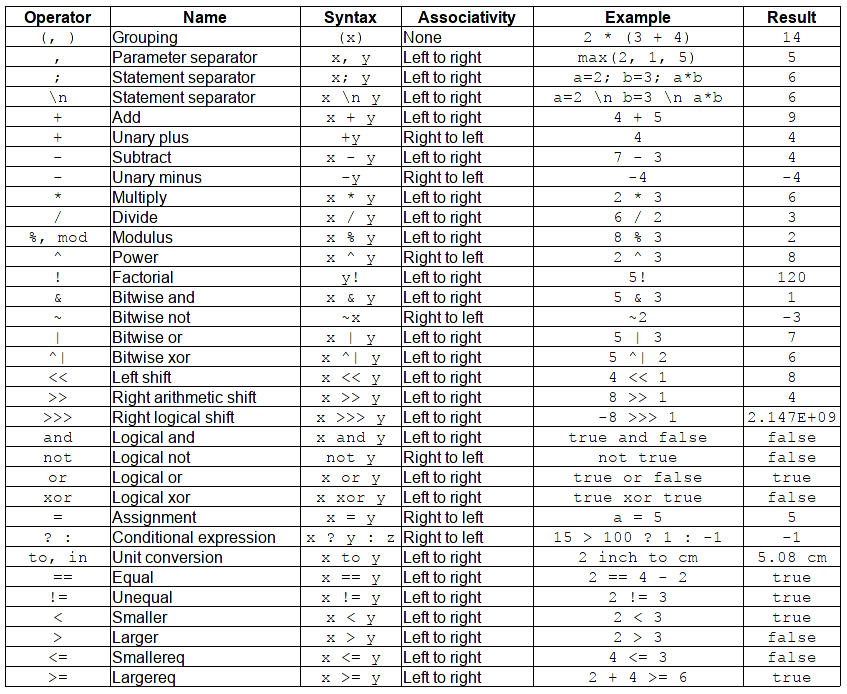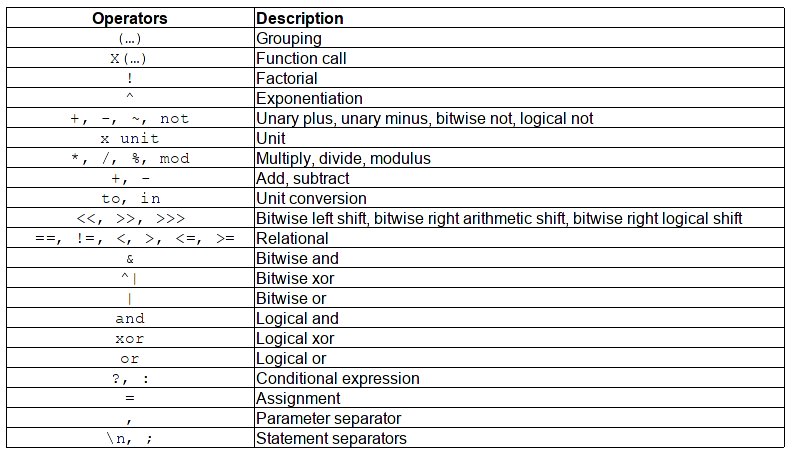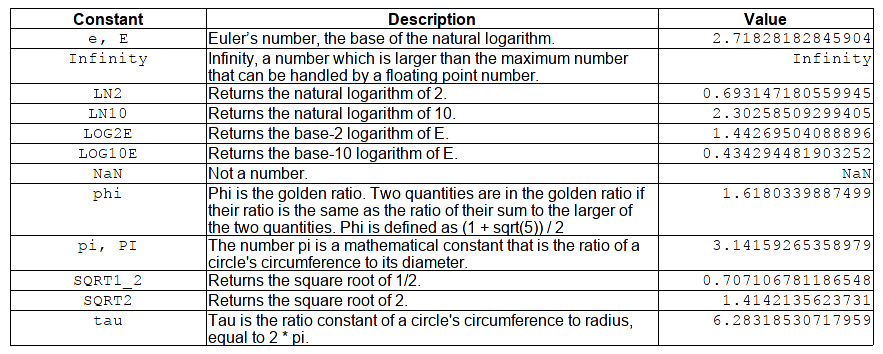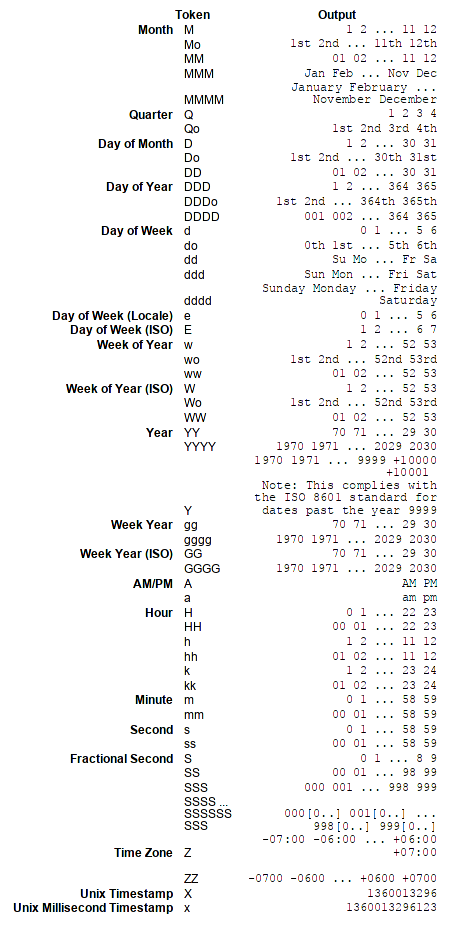• # Formulas

• ## Usage

Pakker includes a full featured calculation engine which let you add automatically calculated values based on the data stored in the card. As an example, if you can define an order card with different items including the related prices and calculated total fields, VAT value and a net total sum.

Input fields, date/time pickers, toggles and rating fields components have a formula option in their respective settings where you can enter formulas. See the syntax section below on how to define formulas.

Pakker uses a special \$() function to retrieve the values of the fields. To retrieve the value of a card component, simply pass its identifier between double quotes to the \$() function. Example: \$("input-1").### Template View with Formulas

The price if each item row is automatically calculated as the multiplication of the unit price and the quantity. In this example, for item row 1, the unit price field has the identifier "up01" and the quantity field the identifier "q01". The item price is then automatically calculated as \$("up01") * \$("q01").

In the database designer, formulas are displayed on each component. This helps you see which input are automatically calculated.### Card View with Calculated Fields

Components with calculated value are always read-only. All calculated fields are automatically updated when the user updates a dependent input. In this example, changing the quantity or the unit price of any item will immediately update the totals and the VAT field.

• ## Syntax

The syntax of Pakker formulas is similar to most calculators and mathematical applications. Parts of a formula are:

• Numbers written with a dot decimal separator and without thousand separator
• Text defined by the enclosing double quotes
• Operators (like +, -, *, ...)
• Functions
• Constants (e.g. Pi)
Formulas can be very simple to very complex, using variables and advanced functions. Example of valid functions :
• `12 / (2.3 + 0.7)`
• `sin( \$("var-a") )`
• `textConcat("Hi ", "there!")`
• a = 1 + 3; b = a * 2; b

### Numbers

Numbers can be expressed as simple decimal numbers or in scientific notation. Example of valid numbers:

• 1.5
• 0.45
• .67
• 1234.56
• 23e10
Please note that results will always be displayed in standard decimal numbers.

### Texts

Text values are always expressed between double quotes ("). If you need to insert a double quote in a value, use the backslash escaped double quote (\"). Example of valid texts:

• "Hi there!"
• "She said \"Hi!\" and came in."
• "1234"

### Booleans

Boolean values represent On/Off, True/False, Yes/No values. Boolean values are used for example in comparison operators or as value of toggle components. Valid values are:

• True
• False

### Operators

Pakker formulas contain all required operators to build easy to complex operators. The table below lists all available operators:### Precedence

Precedence rules are used to determine the order how apply the operators when chained. For example, 2 + 3 * 4 may be interpreted as (2 + 3) * 4 = 20 or 2 + (3 * 4) = 14. The operators have the following precedence, from highest to lowest:### Functions

Functions are called by entering their name, followed by zero or more arguments enclosed by parentheses. The number of arguments depends on the function definition as described below in the functions reference. Function calls can be nested, meaning that a function call can be inserted as a function argument. Example of valid function calls:

• `sqrt(25)`
• `log(1000, 3 + 7)`
• `sin(pi / 4)`
• ```textConcat("Hi ", "there!") ```
• `\$("input-1")`

### Formulas on multiple lines

Formulas can be expressed in several statements. An expression can contain multiple lines, and expressions can be spread over multiple lines. Lines can be separated by a newline character `\n` or by a semicolon `;`. Empty lines are simply ignored. The result of the formula will always be the result of the last statement. Example of valid formulas made of several statements:

• 1 * 3
2 * 3
3 * 3
=> 9
• a=3; b=4; a + b
a * b
=> 12
• a = 2 +
3
=> 5

Comments can be added to explain or describe calculations in the formula. A comment starts with a sharp sign character `#`, and ends at the end of the line. A line can contain a comment only, or can contain an expression followed by a comment. Example of valid comments:

• `# define some variables`
• ```width = 3 ```height = 4 width * height   # calculate the area
• ## Constants

Pakker provides following variables that you can use in your formulas. Example of usage: sin(pi / 4)• ## The \$() Function

#### Getting Values from Components

The \$() function let you retrieve the value of any component of the card. The \$() function accepts one parameter which is the identifier of the component as defined in the component options enclosed by double quotes (example: to retrieve the value from the input field with the identifier input-1, use \$("input-1"). The returned value depends on the component as defined in the following list:

• Label. The text value of the label
• Input Field, type Text. The value of the input field depending on the context of the formula. If the value is a number and used in a mathematical formula, it will be automatically transformed to a number. Otherwise, it will be a text value.
• Input Field Email and URL. The text value of the input field.
• Input Field, type Number. The numeric value of the input field. When the value is used in a text function, the number will be treated as text
• Typewriter. The rich formatted text value as HTML source code
• Datetime Picker. The date value as date
• Select. The selected value as text or number depending on the context of the formula. The Input Field, type Text value usage rules applies to this component
• Toggle. The boolean value of the toggle, true or false
• Rating. The value of the rating as number from 0 to the maximum of the rating component
• Video. The URL of the video
• ## Maths Functions

### Arithmetic functions

• abs(x) Calculates the absolute value of a number.
• crbt(x) Calculates the cubic root of a value
• ceil(x) Round a value towards plus infinity If x is complex, both real and imaginary part are rounded towards plus infinity.
• cube(x) Compute the cube of a value, `x * x * x`.
• divide(x, y) Divide two values, `x / y`.
• exp(x) Calculate the exponent of a value.
• fix(x) Round a value towards zero.
• floor(x) Round a value towards minus infinity.
• gcd(a, b) Calculate the greatest common divisor for two or more values.
• hypot(a, b, ...) Calculate the hypotenusa of a list with values.
• lcm(a, b) Calculate the least common multiple for two or more values
• log(x [, base]) Calculate the logarithm of a value. The base argument is optional. If not provided, the natural logarithm of `x` is calculated. Default value of base is the constant e.
• mod(x, y) Calculates the modulus, the remainder of an integer division.
• multiply(x, y) Multiply two or more values, `x * y`.
• norm(x) Calculate the norm of a number
• nthRoot(a [, root]) Calculate the nth root of a value. The optional parameter root sets the root to be used. The default value: is 2 (Square root).
• pow(x, y) Calculates the power of x to y, `x ^ y`.
• round(x [, n]) Round a value towards the nearest integer. The optional n parameter defines the numbers of decimal in the result. Its default value is 0.
• sign(x) Compute the sign of a value.
• sqrt(x) Calculate the square root of a value.
• square(x) Compute the square of a value, `x * x`.
• subtract(x, y) Subtract two values, `x - y`.
• unaryMinus(x) Inverse the sign of a value, apply a unary minus operation.
• unaryPlus(x) Unary plus operation.
• xgcd(a, b) Calculate the extended greatest common divisor for two values.

### Bitwise Functions

• bitAnd(x, y) Bitwise AND two values, `x & y`.
• bitNot(x, y) Bitwise NOT value, `~x`.
• bitOr(x, y) Bitwise OR two values, `x | y`.
• bitXor(x, y) Bitwise XOR two values, `x ^ y`.
• leftShift(x, y) Bitwise left logical shift of a value x by y number of bits, `x << y`.
• rightArithShift(x, y) Bitwise right arithmetic shift of a value x by y number of bits, `x >> y`.
• rightLogShift(x, y) Bitwise right logical shift of value x by y number of bits, `x >>> y`.

### Logical Functions

• and(x, y) Logical `and`.
• not(x, y) Logical `not`.
• or(x, y) Logical `or`.
• xor(x, y) Logical `yor`.

### Probability Functions

• combinations(n, k) Compute the number of ways of picking `k` unordered outcomes from `n` possibilities.
• factorial(n) Compute the factorial of a value Factorial only supports an integer value as argument.
• gamma(n) Compute the gamma function of a value using Lanczos approximation for small values, and an extended Stirling approximation for large values.
• kldivergence(x, y) Calculate the Kullback-Leibler (KL) divergence between two distributions.
• multinomial(a) Multinomial Coefficients compute the number of ways of picking a1, a2, .
• permutations(n [, k]) Compute the number of ways of obtaining an ordered subset of `k `elements from a set of `n` elements. Permutations only takes integer arguments. The following condition must be enforced: k <= n.
• random([min, max]) Return a random number larger or equal to min and smaller than max using a uniform distribution. The optional min and max parameters are the respectively minimum and maximum boundary for the random value.
• randomInt([min, max]) Return a random integer number larger or equal to `min` and smaller than `max` using a uniform distribution. The optional min and max parameters are the respectively minimum and maximum boundary for the random value.

### Relational Functions

• compare(x, y) Compare two values. Returns 1 when x > y, -1 when x < y, and 0 when x == y. x and y are considered equal when the relative difference between x and y is smaller than epsilon.
• equal(x, y) Test whether two values are equal. The function tests whether the relative difference between x and y is smaller than epsilon.
• larger(x, y) Test whether value x is larger than y. The function returns true when x is larger than y and the relative difference between x and y is larger than epsilon.
• largerEq(x, y) Test whether value x is larger or equal to y. The function returns true when x is larger than y or the relative difference between x and y is smaller than epsilon.
• smaller(x, y) Test whether value x is smaller than y. The function returns true when x is smaller than y and the relative difference between x and y is smaller than epsilon.
• smallerEq(x, y) Test whether value x is smaller or equal to y. The function returns true when x is smaller than y or the relative difference between x and y is smaller than epsilon.
• unequal(x, y) Test whether two values are unequal. The function tests whether the relative difference between x and y is larger than epsilon.

Please note that relational function cannot be used to compare values smaller than approximately 2.22e-16.

### Statistics Functions

• mad(a, b, c, …) Compute the median absolute deviation of a list with values. The median absolute deviation is defined as the median of the absolute deviations from the median.
• max(a, b, c, …) Compute the maximum value of a list with values.
• mean(a, b, c, …) Compute the mean value of a list with values.
• median(a, b, c, …) Compute the median of a matrix or a list with values. The values are sorted and the middle value is returned. In case of an even number of values, the average of the two middle values is returned.
• min(a, b, c, …) Compute the maximum value of a list of values.
• prod(a, b, c, …) Compute the product of a list with values.
• std(a, b, c, …) Compute the standard deviation of a list with values. The standard deviations is defined as the square root of the variance: `std(a, b, c, ...) = sqrt(var(a, b, c, ...))`.
• var(a, b, c, …) Compute the variance of a matrix or a list with values.

### Trigonometry Functions

• acos(x) Calculate the inverse cosine of a value.
• acosh(x) Calculate the hyperbolic arccos of a value, defined as `acosh(x) = ln(sqrt(x^2 - 1) + x)`.
• acot(x) Calculate the inverse cotangent of a value, defined as `acot(x) = atan(1/x)`.
• acoth(x) Calculate the hyperbolic arccotangent of a value, defined as `acoth(x) = atanh(1/x) = (ln((x+1)/x) + ln(x/(x-1))) / 2`.
• acsc(x) Calculate the inverse cosecant of a value, defined as `acsc(x) = asin(1/x)`.
• acsch(x) Calculate the hyperbolic arccosecant of a value, defined as `acsch(x) = asinh(1/x) = ln(1/x + sqrt(1/x^2 + 1))`.
• asec(x) Calculate the inverse secant of a value.
• asech(x) Calculate the hyperbolic arcsecant of a value, defined as `asech(x) = acosh(1/x) = ln(sqrt(1/x^2 - 1) + 1/x)`.
• asin(x) Calculate the inverse sine of a value.
• asinh(x) Calculate the hyperbolic arcsine of a value, defined as `asinh(x) = ln(x + sqrt(x^2 + 1))`.
• atan(x) Calculate the inverse tangent of a value.
• atan2(y, x) Calculate the inverse tangent function with two arguments, y/x.
• atanh(x) Calculate the hyperbolic arctangent of a value, defined as `atanh(x) = ln((1 + x)/(1 - x)) / 2`.
• cos(x) Calculate the cosine of a value.
• cosh(x) Calculate the hyperbolic cosine of a value, defined as `cosh(x) = 1/2 * (exp(x) + exp(-x))`.
• cot(x) Calculate the cotangent of a value.
• coth(x) Calculate the hyperbolic cotangent of a value, defined as `coth(x) = 1 / tanh(x)`.
• csc(x) Calculate the cosecant of a value, defined as `csc(x) = 1/sin(x)`.
• csch(x) Calculate the hyperbolic cosecant of a value, defined as `csch(x) = 1 / sinh(x)`.
• sec(x) Calculate the secant of a value, defined as `sec(x) = 1/cos(x)`.
• sech(x) Calculate the hyperbolic secant of a value, defined as `sech(x) = 1 / cosh(x)`.
• sin(x) Calculate the sine of a value.
• sinh(x) Calculate the hyperbolic sine of a value, defined as `sinh(x) = 1/2 * (exp(x) - exp(-x))`.
• tan(x) Calculate the tangent of a value.
• tanh(x) Calculate the hyperbolic tangent of a value, defined as `tanh(x) = (exp(2 * x) - 1) / (exp(2 * x) + 1)`.

### Unit Functions

• to(x, unit) Change the unit of a value.
• ## Date Functions

• date(year, month, day) Return a date on the given year, month and day. Months are in the range 1-12 (January = 1, December = 12)
• day(date) Return the day in the month of the date
• days(endDate, startDate) Computes the number of days between the start date and the end date
• days360(startDate, endDate, method) Calculate the number of days between start date and the end date on a 360-day year (twelve 30-day months), which is used in some accounting calculations. A logical value that specifies whether to use the U.S. (false) or European (true) method in the calculation.
• dateAdd(date, amount, timeUnit) Return a date which is the given date forwarded by the amount of time unit
• dateSub(date, amount, timeUnit) Return a date which is the given date reversed by the amount of of time unit
• dateDif(endDate, startDate, timeUnit) Compute the difference between the start and end date in the provided time unit
• dateFormat(date, format) Format a date using a formatting pattern. See the table below for formatting options
• hour(date) Return the hour of the given date
• minute(date) Return the minute of the given date
• month(date) Return the month of the given date. The value is 0-based (0 = January, 11 = December)
• now() Return the current date at the time it was called
• second(date) Return the second of the given date
• weekday(date) Return the weekday of the given date. Weekdays values are 0 = Sunday, 1 = Monday, ..., Saturday = 6
• isoWeekday(date) Return the ISO weekday of the given date. Weekdays values are 1 = Monday, 2 = Tuesday, ..., Sunday = 7
• weekNum(date) Return the week number. The first week of the year is the one containing the 1st of January
• isoWeekNum(date) Return the ISO week number. The first week of the year is the one having at least 4 dates in the date year. Example: if the 1st of January is a Friday, the first week of the year will start on the 4th of January.
• year(date) Return the year of the given date
• yearFrac(startDate, endDate) Return the number of years between the start and end date

Time units
Available time units used in the date functions are seconds (s), minutes (m), hours (h), days(d), months (M), years (y)

Date Patterns
Date patterns are defined as follows:• ## Text Functions

• textConcat(a, b, ...)  Return the concatenation of all given texts
• textLength(a) Return the length of the text
• textCharAt(a, position) Return the character position of the text a at the 0-based position. The first character of the text is at position 0
• textStartsWith(a, prefix) Test whether the text value a starts with the prefix
• textEndsWith(a, suffix) Test whether the text a ends with the suffix.
• textIndexOf(a, search) Return the index of the search text in the text a. If the value is not found, the function returns -1
• textSubstring(a, from, to) Return the portion of the text a between positions from and to. The positions are 0-based
• textToLowerCase(a) Return the text a with all letters transformed to lowercase
• textToUpperCase(a) Return the text a with all letters transformed to uppercase
• textTrim(a) Return the text a after removing all whitespaces characters at the beginning and end of the text. Are considered as whitespace the spacetabline feedreturn characters
• ## Utility Functions

• isInteger(x) Test whether a value is an integer number.
• isNaN(x) Test whether a value is NaN (not a number).
• isNegative(x) Test whether a value is negative: smaller than zero.
• isNumeric(x) Test whether a value is an numeric value.
• isPositive(x) Test whether a value is positive: larger than zero.
• isPrime(x) Test whether a value is prime: has no divisors other than itself and one.
• isZero(x) Test whether a value is zero.